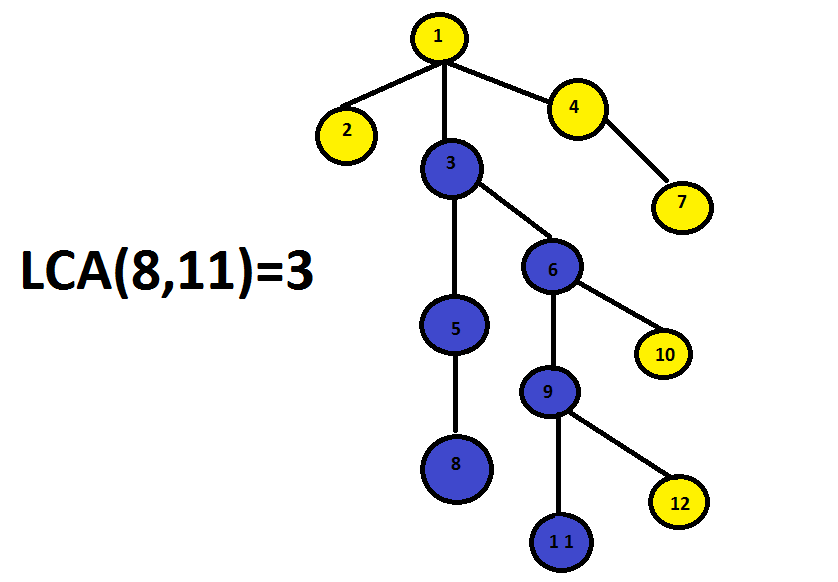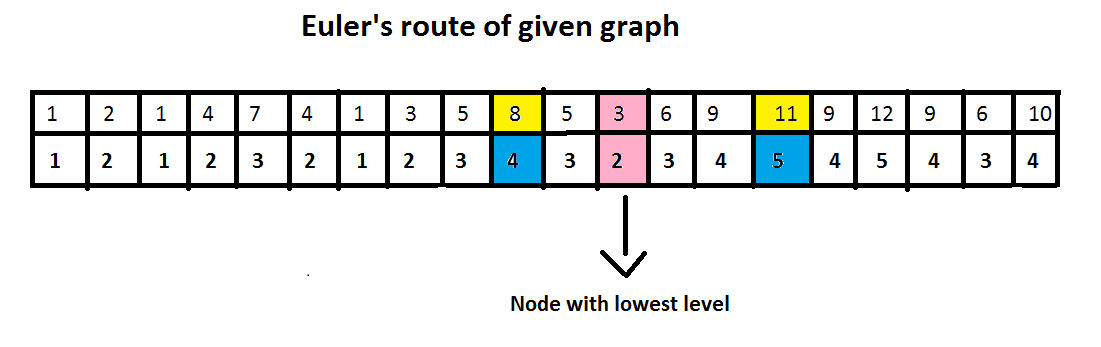×

Search anything:

# Using Farach Colton and Bender Algorithm to solve LCA【O(V) query】

#### Algorithms Graph Algorithms lowest common ancestorFor a query of the form (u,v) on a tree G we have to find the Lowest Common Ancestor of nodes u and v. The Lowest Common Ancestor of nodes and in a tree is the shared ancestor of and that is located farthest from the root.It is obvious that the lowest common ancestor of vertices u and v lies on the shortest path from u to v. Specifically, for example, if u is the ancestor v, u is then their lowest common ancestor.

## Basic Idea:

Traverse all the nodes using the Depth First Search graph traversal and keep a record of all the visited nodes and there corresponding heights. This is also called as the Euler tour of the tree.

Now suppose we have a query to find the lowest common ancestor of u,v.

Consider the vertices that we visit in the Euler tour between the first visit of u and the first visit of v.

It is easy to see, that the LCA(u,v) is the vertex with the lowest height on this path. The LCA has to be the part of the shortest path between (u,v).

## Example:

Given a tree G ,we have to find the lowest common ancestor in the path from (u,v)=(8,11)To solve this we maintain two arrays to:

• record the euler route
• other to record the corresponding levels of the nodes• The first row denotes the order of vertices that will appear in a depth first traversal

• The second row denotes the level or depth of the vertex just above it

If we do the dfs traversal of the tree from the root node 1->2 then from the leaf node 2 we will return to node 1 and explore it's children again.Now we will do the traversal 3->5->8 and then return through the same path to node 3 and explore its children till the leaf nodes and process is carried recursively,till we traverse the whole tree and as we traverse each node we will maintain an array sidewise which will store the level of corresponding node as shown in the figure.

After this to find the Lowest Common Ancestor we find the first occurrence of given numbers in the euler route and then thus the node with lowest level in the range, which will be the answer.

## Time complexity-:

Time complexity for preprocessing is O(V+E) as we use bfs and dfs approach for finding level of each node and euler path respectively where V and E are the number of vertices and edges in the given tree respectively.

Queries can be answered with O(V) time complexity where V is the number of nodes in the given tree.

You will be able to understand it better after going through the C++ implementation.

## Pseudo Code-:

There are two functions through which we implement LCA problem

• bfs: This function is used to calculate the level of each node using the bfs graph traversal.

Pseudocode of BFS:

``````void bfs(arguments)
{
queue<ll>q // data structure for implementing bfs
q.push(root) // root node pushed in the queue
level[root]=0 // level of root node set as '0'
while(queue not empty)
{
t=q.front() // storing front of queue
q.pop() // popping front of queue
flag=0 // flag reset for incoming nodes in the queue
for(i=0;i<adj[t].size();i++) // accessing children of popped node
{

{
if(flag==0)
{
l++;
flag++; // flag set so that same level is designated to nodes at same height
}

level[adj[t][i]]=l; // level of node just pushed into the queue
}
}
}
``````
• euler_path-This function stores the euler tour of the graph in an array 'euler'.This function is implemented in the same way as dfs graph traversal with some minor changes.

1 Store the node passed as argument to the function 'euler_path' in the 'euler' array and then recursively call the function till the leaf node occurs durig dfs traversal.

2 As the leaf node occurs track back to the parent and store its parent in the 'euler' array and explore its parent for any unvisited nodes.If there are any then visit it and explore its children again.

Pseudocode:

``````counter=0;//global variable
void euler_path(arguments)
{
visited[i]=1 // mark node as visited
euler[counter]=i // store node in euler path
counter++;

{
if(visited[adj[i][j]]==-1) //if the is not yet visited
{
euler_path(arguments) //go into recursive function to access children of given node i.e. dfs approach
}
}
euler[counter]=parent //bactracked ancestor stored in 'euler' array
counter++
}
``````

## C++ Implementation-:

``````#include <bits/stdc++.h>
using namespace std;
#define ll long long int
ll counter=0;//Global variable set as counter for 'euler' array
void euler_path(ll i,vector<ll>adj[],ll visited[],ll euler[],ll parent)
{
ll j;
visited[i]=1;
euler[counter]=i;//visited node stored in 'euler'
counter++;

{

{

}

}
euler[counter]=parent;//backtracked parent stored in 'euler'
counter++;

}
{
ll l=0,t,i,flag=0;
queue<ll>q;
q.push(root);
level[root]=l;//level of root node
while(!q.empty())
{
t=q.front();
q.pop();
flag=0;
{

{
if(flag==0)
{
l++;
flag++;
}

level[adj[t][i]]=l;//level of node just pushed into the queue
}
}
}
}
int main()
{
ll t,n;
cin>>t;
while(t--)
{
cin>>n;
ll level[n+1],k,j,i,c,q,x,y;//'level' array stores the level of each node
ll visited[n+1];//'visited' array tells whether a particular node is visited or not on DFS traversal
ll euler;
ll firstvisit[n+1];//stores the first index of a node in euler path
memset(level,-1,sizeof(level));
memset(visited,-1,sizeof(visited));
memset(firstvisit,-1,sizeof(firstvisit));
ll min=-1,lca,hl;
for(i=1;i<=n;i++)
{
cin>>k;
for(j=1;j<=k;j++)
{
cin>>c;
}
}
bfs(level,adj,1);//To store the level of all the nodes in 'level' array
euler_path(1,adj,visited,euler,-1);//To store the euler path in 'euler' array

for(i=0;i<counter-1;i++)
{
if(firstvisit[euler[i]]==-1)
{
firstvisit[euler[i]]=i;
}
}

/*PROCESSING QUERIES*/
cin>>q;
for(i=1;i<=q;i++)
{
min=INT_MAX;
cin>>x>>y;

for(j=firstvisit[x];j<firstvisit[y];j++)
{
if(level[euler[j]]<min)
{
lca=euler[j];
min=level[euler[j]];
}
}

cout<<lca<<"\n";
}

}
return 0;
}
``````

Input Statement

The first line of input will be the number of test cases. Each test case will start with a number N the number of nodes in the tree. Nodes are numbered from 1 to N. The next N lines each one will start with a number M the number of child nodes of the Nth node,followed by M numbers the child nodes of the Nth node. The next line will be a number Q the number of queries you have to answer for the given tree T. The next Q lines each one will have two number v and w in which you have to find the LCA of v and w in T.

Input will guarantee that there is only one root and no cycles.

### Input-:

``````1
7
3 2 3 4
0
3 5 6 7
0
0
0
0
2
5 7
2 7
``````

### Output

``````Case 1:
3
1
``````

### Other Techniques-:

• Square Root Decomposition-It is possible to obtain a solution answering each query in O(sqrt(n)) with preprocessing in O(n) time where n is the number of nodes in the given tree.

• Segment Tree-It is possible to obtain a solution answering each query in O(log(n)) with preprocessing in O(n) time where n is the number of nodes in the given tree.

### Question-:

Implement the Lowest Common Ancestor problem using Square root decomposition method.#### Siddharth Agarwal

Intern at OpenGenus | Bachelor of Technology (2017 to 2021) in Computer Science at Institute of Engineering and Technology

Using Farach Colton and Bender Algorithm to solve LCA【O(V) query】